# Design a four-way, 3-bit multiplexer that use only NOR gates. (5%) 4, Design a four-way, 3-bit multiplexer tha...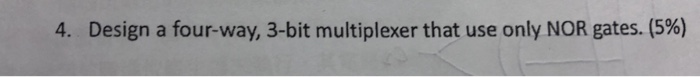Design a four-way, 3-bit multiplexer that use only NOR gates. (5%) 4,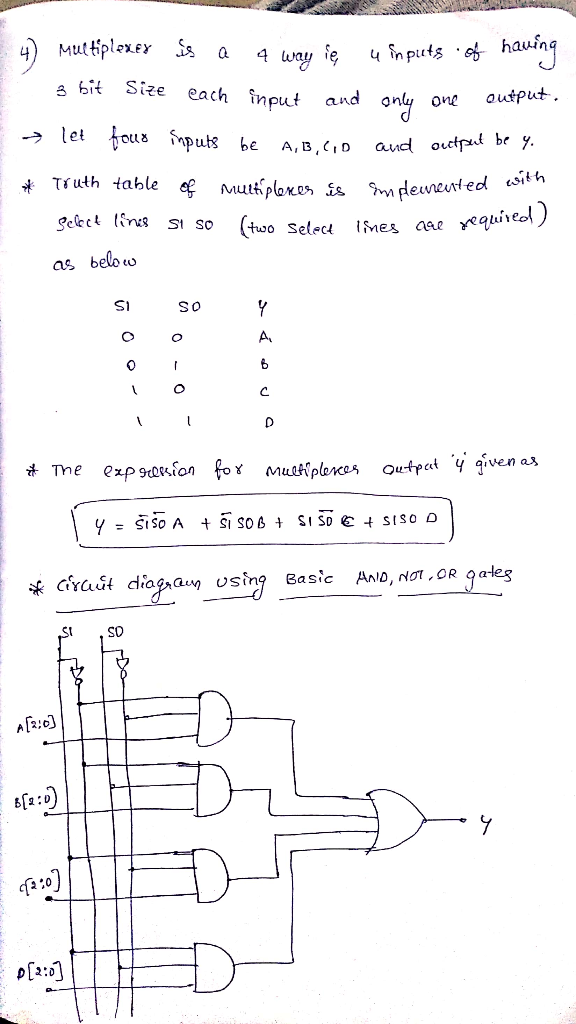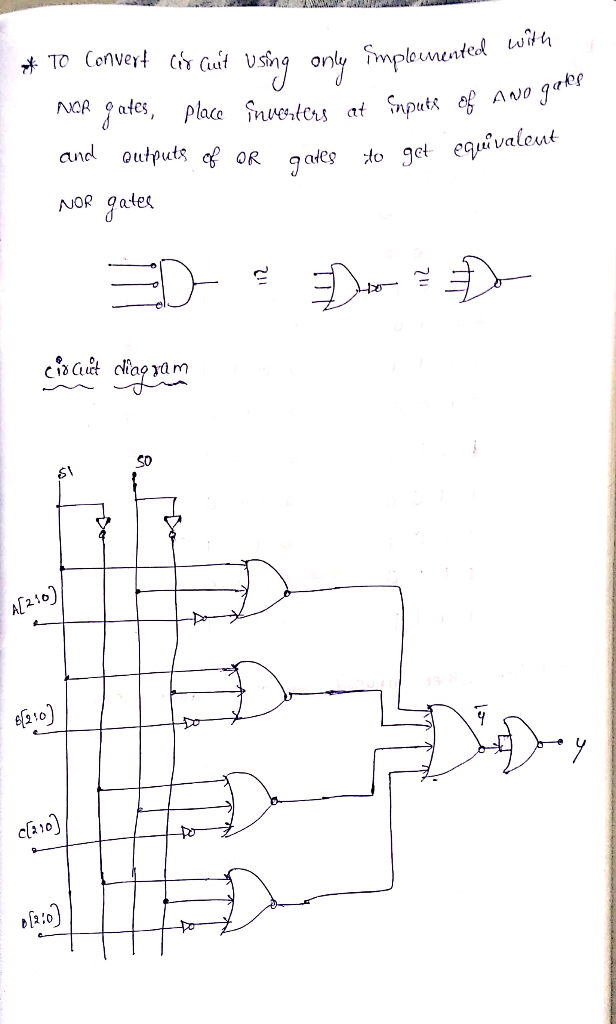##### Add Answer of: Design a four-way, 3-bit multiplexer that use only NOR gates. (5%) 4, Design a four-way, 3-bit multiplexer tha...
Similar Homework Help Questions
• ### design the circuit using only nor gatesDesign the abovecircuit only using nor gates

• ### Use as few 3-input NOR gates as possible to design a bubble detector circuit for 8-bit thermomete...

Use as few 3-input NOR gates as possible to design a bubble detector circuit for 8-bit thermometer code. An n-bit thermometer code represents an integer m, with m 1s followed by (n-m) 0s. 1-bit bubble is an error in coding when a solitary 0 (or 1) is found in between two 1s (or 0s). What is the size of your circuit in terms of the number of NOR gates used? Give a gate level schematic diagram for your circuit. Implement...

• ### Tim Question 1 Atte 20 pts 2H 24 Design a 1-bit Full Adder using NOR gates...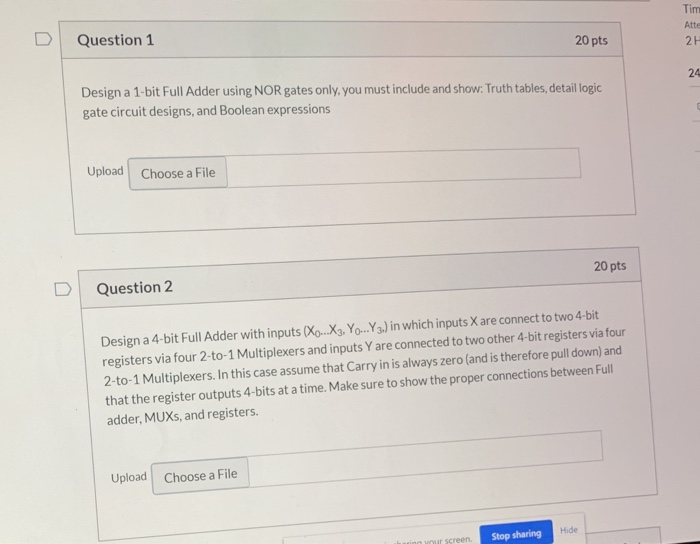Tim Question 1 Atte 20 pts 2H 24 Design a 1-bit Full Adder using NOR gates only, you must include and show: Truth tables, detail logic gate circuit designs, and Boolean expressions Upload Choose a File 20 pts Question 2 Design a 4-bit Full Adder with inputs (Xo...X3, Yo...Y3) in which inputs X are connect to two 4-bit registers via four 2-to-1 Multiplexers and inputs Y are connected to two other 4-bit registers via four 2-to-1 Multiplexers. In this case...

• ### Design 5 seconds. Use 8-bit counter and logic gates for your hardware design Assume clock frequency...Design 5 seconds. Use 8-bit counter and logic gates for your hardware design Assume clock frequency of 80 Hz for the 8-bit counters that are used in the design Show all work and provide the logic diagram for full credit Watchdog-Timer that will generate an overflow (interrupt) output every a Design 5 seconds. Use 8-bit counter and logic gates for your hardware design Assume clock frequency of 80 Hz for the 8-bit counters that are used in the design Show...

• ### Write out the truth table for an XOR function. Then using only NOR gates design a...

Write out the truth table for an XOR function. Then using only NOR gates design a gating circut to perform the XOR Function. (Hint: The XOR function is just the Not OR function… start by writing out the OR truth table.)

• ### Create 16:4 multiplexer.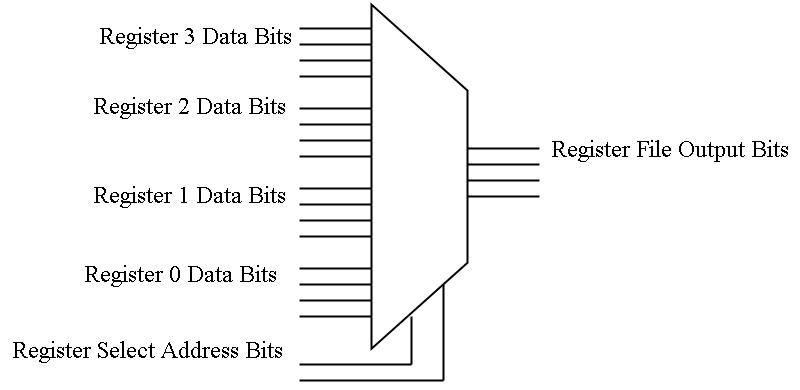Design is a 4-bit, 4:1 multiplexer which selects among 4 groups of 4-bit data inputs. Use standard 74xxx family parts showing the proper connections.The symbol for the 16:4 multiplexer looks as follows:

• ### Design 3- to – 8 decoder using logic gates with enabler, AND, NOT, etc..? Design 3- to – 8 decode...

Design 3- to – 8 decoder using logic gates with enabler, AND, NOT, etc..? Design 3- to – 8 decoder using only two 2-to-4 decoders graphical blocks, use enabler input? a) Design a 3-bit ripple-carry adder using AND, OR, NOT, EXOR, etc.; include carry-in (Cin), carry-out (Cout) and overflow input/output signals? Note: Design for 1-bit first, then extrapolate to 4-bit using 1-bit full-adder graphical block. Design a 3-bit ripple-carry subtractor using AND, OR, NOT, EXOR, etc..; include carry-in (Cin), carry-out...

• ### Use a multiplexer to implement a 4-bit (unsigned) prime number detector.

Use a multiplexer to implement a 4-bit (unsigned) prime number detector.

• ### I need circuit not code. Thank you Use of JK-MS-FFs and logic gates to design of...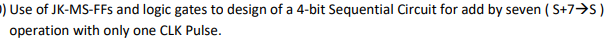I need circuit not code. Thank you Use of JK-MS-FFs and logic gates to design of a 4-bit Sequential Circuit for add by seven ( S+7 S) operation with only one CLK Pulse Use of JK-MS-FFs and logic gates to design of a 4-bit Sequential Circuit for add by seven ( S+7 S) operation with only one CLK Pulse

• ### NOR decoder design problem

Using only inverters and NOR gates (and only a minimum number of these), show the design of a 2:4 decoderwhose output lines are low when inactive. Assume the input enable is active high. Be sure to use the convention that theactive line is the one whose subscript is the decimal equivalent of the applied binary address.

Need Online Homework Help?